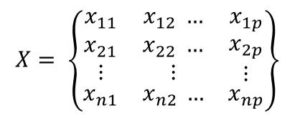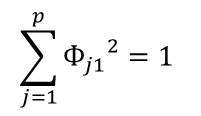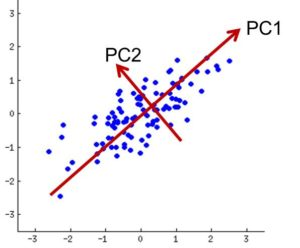# PCA Tutorial 2 – How to Perform Principal Components Analysis (PCA)

Now you know some theories about Principal Components Analysis (PCA) and now we are going to go through how to actually perform it.Next we would take a live dataset and actually perform PCA using R.

As a brief introduction, keep in mind that the principal components are obtained by matrix multiplication. Precisely, we are would have to multiply the original data matrix X with loadings matrix Φ. This would give us the scores. So you can jump to About Scores and Loadings, and then come back to  continue

1. The Second Principal Component (Z2)

We have out data X  which is n x p dataset. Which means n observations (records or rows) and p features(or variable). This is represented as shown in X matrix.We define the first principal components of a set of features X1, X2, . . . , Xp as the normalized linear combination of the features/variables in the original data:

Z1 = Φ11X1 + Φ21X2 + . . . + Φp1Xp    ——-      (PC1)

that has the highest variance.

The elements Φ are called the loadings of the first principal component and they are such that the sum of square of the Φs equal to 1 as in;The combinations of the loadings make up the principal components loading vector (Φ11 Ф21 . . . Фp1)T and the combination of the loadings vector make up the loadings matrix.

X1, X2, . . . , Xp are normalized linear combination of the feature variables which means that they have a mean equal to zero and a standard deviation equal to one.

2. The Second Principal Component (Z2)

The second principal component Z2 is also a linear combination of the predictor variables (features) that has has the second-highest variance following Z1.  It should also be noted the Z2 is uncorrelated with Z1 and that means that the direction of Z1 and Z2 are orthogonal as shown in Figure 1.0.

Z2 = Φ12X1 + Φ22X2 + . . . + Φp2Xp    ——-      (PC1)

Additional Components can be computed in a similar manner.

Once we are done computing the principal components say, (Z1 and Z2) we can then plot them on a plane one against the other on a 2d plot. The output is similar to Figure 1.0Figure 1.0: PC1 and PC2 (Z1 and Z2) are orthogonal to each other

In PCA we kind of break down the original data matrix X and break it down into item:

• set of eigen values, λ
• set of eigen vectors, Φ

These two together make up the eigen pair. Now let’s picture what the set of eigen vector would look like. This is shown in the matrix below and this is called the loadings matrix.

• this matrix is a square matrix ( has same rows and columns)
• the dimension of this matrix (p x p) is comes from the number of features of the original matrix X

Each column of the loadings matrix corresponds to one principal component.

for the first column, we have the following elements  (Ф11 Ф21 . . . Фp1). This is the loadings of the first principal component

for the second column, we have the following elements (Ф12 Ф22 . . . Фp2). This is the loadings of the second principal component

for the third column, we have the following elements (Ф13 Ф23 . . . Фp3). This is the loadings of the third principal component

for the pth column, we have the following elements (Ф1p Ф2p . . . Фpp).. This is the loadings of the pth principal components

Now let’s attempt to multiply the matrix X and  Ф and let’s see what we would obtain. Let’s call this matrix. This operation gives us a set of numbers called the scores (just a set of 1 dimensional values)

X is n x p matrix
Ф is p x p matrix

Therefore, the multiplying X by Ф would give us just a one dimensional data. If you are not sure of this you can go through a Brief Review of Matrix Operations made especially for this tutorial.

The product of the two matrices would give us the following elements:

Taking the first row of X and  the first column of Ф, we would have:

x11Ф11 + x12Ф21 + . . . + x1pФp1 (this is the first principal component (PC1)

Taking the second row of X and  the second column of Ф, we would have:

x21Ф12 + x22Ф22 + . . . + x2pФp2 (this is the second principal component (PC2)

And so on

4. Interpreting Principal Components

When we perform PCA on our n x p dataset, we obtain a loadings matrix Φ which is also an n x p matrix.  We can then use just the first few columns(say 2 columns) of this matrix as our principal components  and project our data along these directions.

When we perform PCA, we are projecting the data from  p-dimensional space into two dimensional space

What does all this mean. Let’s illustration by using a particular dataset. This would help us to see how our dataset is affected after performing PCA on the data.

Now that you understand how to perform Principal Components Analysis, let’s now move a similar topic which is Singular Value Decomposition.#### kindsonthegenius

Kindson Munonye is currently completing his doctoral program in Software Engineering in Budapest University of Technology and Economics

View all posts by kindsonthegenius →